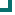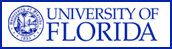Streptococcus pneumoniae (NORTHEAST Data) 2004 2005 2006 2007 2008 2009 2010 penicillin 71.2%n=1265 60.9%n=593 61.8%n=793 64.2%n=689 75.5%n=424 75.5%n=147 amoxicillin/clavulanate 86.8%n=91 81.3%n=16 82.4%n=34 100%n=24 ampicillin 100%n=27 vancomycin 100%n=1043 99.8%n=548 100%n=622 99.9%n=736 99.8%n=452 99.5%n=216 cefuroxime 82.2%n=135 78%n=82 75.4%n=114 75.5%n=98 cefotaxime 94.8%n=154 88.5%n=157 92.4%n=131 91.3%n=161 92.6%n=68 ceftriaxone 97.7%n=1097 96.5%n=538 96.4%n=646 96.1%n=623 96.3%n=465 96.5%n=171 clindamycin 77.2%n=136 75.2%n=121 73%n=141 86.9%n=84 81.6%n=114 erythromycin 73.4%n=726 61%n=492 64.3%n=686 67.8%n=512 66.6%n=365 78.3%n=83 azithromycin 54.4%n=125 46.4%n=181 46%n=100 58.6%n=29 73%n=37 clarithromycin 59%n=100 31.3%n=16 ciprofloxacin 96.8%n=31 ofloxacin 92.7%n=41 97.9%n=48 levofloxacin 98.8%n=409 98.8%n=345 98.1%n=263 99.1%n=331 98.1%n=315 98.1%n=159 gatifloxacin 100%n=103 100%n=160 100%n=100 moxifloxacin 99.2%n=484 98%n=101 98.6%n=351 98.1%n=160 100%n=44 TMP/SMX 76.7%n=206 75.6%n=315 72.8%n=276 79.2%n=355 82.1%n=156 85.2%n=81 chloramphenicol 92.5%n=120 90.5%n=137 95.9%n=246 97.9%n=194 100%n=24 Totals 86.8%n=6235 81.4%n=3834 82.2%n=4485 85.7%n=4035 87.3%n=2480 90.4%n=894

This data is accurate as of: 11/22/2016 11:53:35 AM

Drugs with less than 10 isolates are removed from the chart and from the graph. The following drugs had some data removed: amoxicillin/clavulanate, ampicillin, ofloxacin, cefuroxime, clarithromycin, clindamycin, ciprofloxacin, cefotaxime, azithromycin, moxifloxacin, chloramphenicol, erythromycin, TMP/SMX.ARMProgram.com is a project run by the University of Florida. Copyright 2011. All Rights Reserved. Site by JMarc Technologies, LLC